Next: Multi-Function Variation Up: Hamiltonian Dynamics Previous: Calculus of Variations

Conditional Variation

Suppose that we wish to find the functionwhich maximizes or minimizes the functional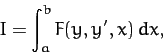(691)

subject to the constraint that the value of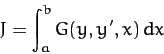(692)

remains constant. We can achieve our goal by finding an extremum of the new functional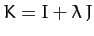, where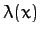is an undetermined function. We know that, since the value of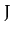is fixed, so if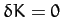then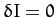as well. In other words, finding an extremum ofis equivalent to finding an extremum of. Application of the Euler-Lagrange equation yields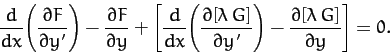(693)

In principle, the above equation, together with the constraint (692), yields the functionsand. Incidentally,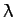is generally termed a Lagrange multiplier. Ifand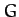have no explicit-dependence thenis usually a constant.

As an example, consider the following famous problem. Suppose that a uniform chain of fixed lengthis suspended by its ends from two equal-height fixed points which are a distance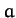apart, where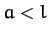. What is the equilibrium configuration of the chain?

Suppose that the chain has the uniform density per unit length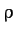. Let the- and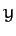-axes be horizontal and vertical, respectively, and let the two ends of the chain lie at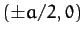. The equilibrium configuration of the chain is specified by the function, for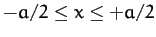, whereis the vertical distance of the chain below its end points at horizontal position. Of course,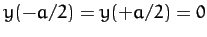.

According to the discussion in Section 3.2, the stable equilibrium state of a conservative dynamical system is one which minimizes the system's potential energy. Now, the potential energy of the chain is written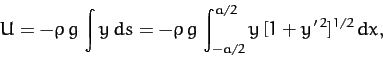(694)

where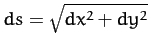is an element of length along the chain, and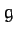is the acceleration due to gravity. Hence, we need to minimize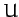with respect to small variations in. However, the variations inmust be such as to conserve the fixed length of the chain. Hence, our minimization procedure is subject to the constraint that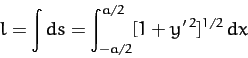(695)

remains constant.

It follows, from the above discussion, that we need to minimize the functional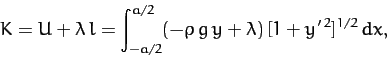(696)

whereis an, as yet, undetermined constant. Since the integrand in the functional does not depend explicitly on, we have from Equation (688) that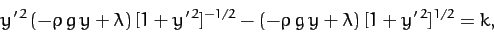(697)

where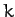is a constant. This expression reduces to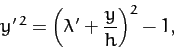(698)

where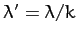, and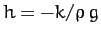.

Let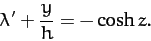(699)

Making this substitution, Equation (698) yields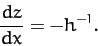(700)

Hence,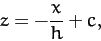(701)

where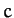is a constant. It follows from Equation (699) that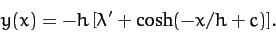(702)

The above solution contains three undetermined constants,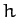,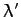, and. We can eliminate two of these constants by application of the boundary conditions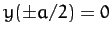. This yields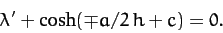(703)

Hence,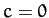, and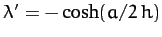. It follows that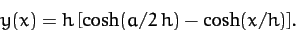(704)

The final unknown constant,, is determined via the application of the constraint (695). Thus,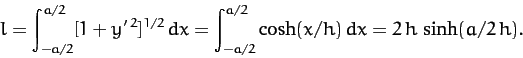(705)

Hence, the equilibrium configuration of the chain is given by the curve (704), which is known as a catenary, where the parametersatisfies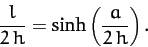(706)Next: Multi-Function Variation Up: Hamiltonian Dynamics Previous: Calculus of Variations
Richard Fitzpatrick 2011-03-31